Thursday 09th July 2020

CBSE Guess > Papers > Question Papers > Class XII > 2006 > Chemistry > Compartment Outside Delhi Set - I

Chemistry — 2006 (Set I — Compartment Outside Delhi)

General Instructions: See in the Set - I, D.B., 2006 (Comptt.)

Q. 1. State Raoult’s law for solutions of nonvolatile solutes. (1)

Q. 2. What makes the crystal of KCI appear sometimes violet? (1)

Q. 3. Define activation energy of a reaction. (1)

Q. 4. Give the IUPAC name of the following compound: (1)

CH3CH (NO2) CH2CH3

Q. 5. Complete the reaction equation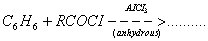.

Q. 6. On what basis can you say that in an atom an s-orbital is spherical and the p-orbitals are dumb-bell shaped? (2)

Q. 7. Prove that in a process heat change at constant pressure is a measure of the change in enthalpy. (2)

Q. 8. What happens when

1. Tin dissolves in a hot alkali solution?
2. Xe03 reacts with an aqueous alkali solution?
Write the complete chemical equation in each case. (2)

Or

Write balanced chemical equations for the following processes

1. SiO2 is treated with an excess of KOH.
2. Bi2O3 is treated with conc. HNO3.

Q. 9. How is the variability in oxidation states of the tansition elements different from that of the non-transition elements? Illustrate with examples. (2)

Q. 10. Convert the following wedge-and-dash formula to Fischer projection formula. (2)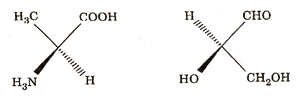Q.11. How are the following conversions carried out (2)

1. Propene to porpan-2-ol
2. Phenol to salicylaldehyde

Q. 12. Differentiate between a homopolymer and a copolymer. Give one example of each. (2)

Q. 13. Compare the relative stability of the following species and indicate their magnetic (diamagnetic or paramagnetic) characters O2 +, O2 and O2 2
Is only bond order a quantitative measure of the bond strength in a species? (3)

Or

Draw the molecular orbital diagrams for the species N2+ and N2-- .Comment on the magnetic property and bond strength of each.

Q. 14. What is a semiconductor? Describe two main types of semiconductors, giving examples and their distinctive features (3).

Q. 15. A 0.2 per cent aqueous solution of a non-volatile solute exerts a vapour pressure of 1.004 bar at 1000 C. What is the molar mass of the solute?
(Given: Vapour pressure of pure water at 100°C is 1.013 bar and molar mass of water is 18 g mol —1 ) (3)

Q. 16. Calculate ∆ r G° and the equilibrium constant for the following reaction at 298 K and 1 atmosphere pressure:
CO (g) + 3 H2 (g) ↔ CH4 (g) + H2 O (l)
(Given that at 298 K ∆ r H° for the reaction is — 250.3 kJ mol --1 and ∆ f S° for the reaction is — 333.3 JK --1 and R = 8.314 J K — 1 mol --1 (3)

Q. 17. At 300° C the thermal dissociation of HI is 20%. What will be the equilibrium concentrations of H2 and l2 in the system H2 + l2 ↔2Hl at this temperature if the equilibrium concentration of HI is found to be 0.96 mol L —1 ? (3)

Q.18. State what you will observe when

1. an electrolyte is added to a colloidal solution,
2. an electric current is passed through a colloidal solution,
3. a beam of light is passed across pure water and then across a colloidal solution of sulphur when you are not in the path of light. (3)

Q.19. How would you account for the following observations: (3)

1. The enthalpies of atomisation of the transition metals are high.
2. Of the lanthanoids only Cerium (Z = 58) is known to exhibit quite stable + 4 state in solutions.
3. Sodium thiosulphate solution is used in the ‘fixing’ step of photography.

Q. 20. Draw the structures of the following: (3)

1. Cis-dichlorotetracyanochromate (III)
2. Pentaamminenitrito-N-cobalt (III)
3. Hexamethyldialuminium

Q. 21. Write the product nucleides for the following radiaction decays: (3)

1.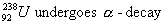2.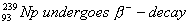3.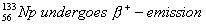(Note: You can put ‘X’ for the symbol if it is not known to you).

Q. 22. How would you bring about the following conversions: (3)

1. Propanal to butanone
2. Benzaldehyde to benzophenone
3. Benzoyl chloride to benzonitrile
Give the complete chemical equation and reaction conditions in each case.

Q. 23.

1. Explain the following observations:
1. Primary amines are higher boiling than comparable tertiary amines.
2. Aromatic amines are less basic than aliphatic amines.
2. Mention two important uses of N, N-Dimethyl aniline (DMA). (3)

Q. 24. Describe the following with examples:

1. Tranquilizers
2. Preservatives
3. Biliquid propellants

Q. 25.

1. State the products of electrolysis obtained on the cathode and the anode in the following cases
1. A dilute solution of H2S04 with platinum electrodes
2. An aqueous solution of AgNO3 with silver electrodes
2. Write the cell formulation and calculate the standard cell potential of the galvanic celL in which the following reaction takes place:
Fe2+ (aq) + Ag+ (aq) → Fe3+ (aq) + Ag (s)
Calcuate ∆ f G° for the above reaction.
[Given: E° Ag+ / Ag = + 0.80 V and E° Fe3+ / Fe2+ = + 0.77 V; F = 96500 C mol —1] (2, 3)

Or

1. What are fuel cells? Write the electrode reactions of a fuel cell which uses the reaction of hydrogen with oxygen.
2. Write the cell formulation and calculate the standard cell potential of the galvanic cell which uses the following reaction in its operation:
2 Cr (s) + 3 Cd2+ (aq) → 2 Cr 3+ (aq) + 3 Cd (s)
Calculate ∆r G0 for the above reaction.
[Given: E0Cr3+ / Cr = — 0.74 V and E0 cd2+ / Cd = — 0.40 V; F = 96500 C mol—1]

Q. 26.

1. How would you account for the following:
1. Tin (II) is a stronger reducing agent than lead (II).
2. Enthalpy of dissociation for F2 is much less than that for Cl2.
3. Sulphur in vapour state exhibits paramagnetism.
2. Draw the structures of the following molecules:
1. H3P02
2. XeOF4

Or

1. Explain the following observations:
1. Anhydrous aluminium chloride has a lower melting point than aluminium fluoride
2. Bismuth oxide is not acidic in any of its reactions
3. HF is weaker acid than HI in aqueous solutions
2. Draw the structures of the following molecules
1. XeF4
2. SF4

Q. 27.

1. Enumerate the structural differences between DNA and RNA. Write down the structure of a nucleoside which is present only in RNA.
2. What are reducing sugars ? Give an example each of a reducing sugar and a non-reducing sugar. (3, 2)

Or

1. Name the three classes of lipids and give one example of each class.
2. Explain the term mutarotation giving an example.

 Chemistry 2006 Question Papers Class XII Delhi Outside Delhi Compartment Delhi Compartment Outside DelhiSet ISet ISet ISet I

 CBSE 2006 Question Papers Class XIIEnglishSociologyFunctional EnglishPsychologyMathematicsPhilosopyPhysicsComputer ScienceChemistryEntrepreneurshipBiologyInformatics PracticesGeographyMultimedia & Web TechnologyEconomicsBiotechnologyBusiness StudiesPhysical EducationAccountancyFine ArtsPolitical ScienceHistoryAgriculture